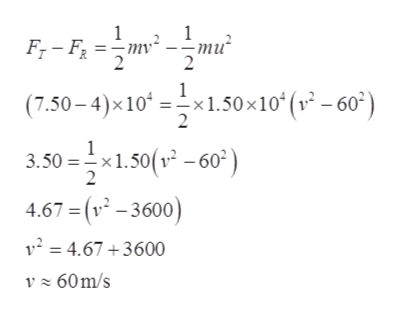# An airplane of mass 1.50 x 104 kg is moving at 60.0 m/s.The pilot then increases the engine’s thrust to 7.50 x 104 N.The resistive force exerted by air on the airplane has a magnitudeof 4.00 x 104 N. (a) Is the work done by the engineon the airplane equal to the change in the airplane’s kineticenergy after it travels through some distance through the air?Is mechanical energy conserved? Explain. (b) Find the speed of the airplane after it has traveled 5.00 x 102 m. Assume theairplane is in level flight throughout the motion.

Question
11 views

An airplane of mass 1.50 x 104 kg is moving at 60.0 m/s.
The pilot then increases the engine’s thrust to 7.50 x 104 N.
The resistive force exerted by air on the airplane has a magnitude
of 4.00 x 104 N. (a) Is the work done by the engine
on the airplane equal to the change in the airplane’s kinetic
energy after it travels through some distance through the air?
Is mechanical energy conserved? Explain. (b) Find the speed of the airplane after it has traveled 5.00 x 102 m. Assume the
airplane is in level flight throughout the motion.

check_circle

Step 1

Mass of the airplane = 1.50 X 104 kg

Initial Speed of the airplane = 60 m/s

Thrust force = 7.50 X 104 N

Resistive force = 4 X 104 N

Distance travelled by the plane = 5 X 102 m

Step 2

Part (a): no, as the work done by net force is equals to the change in the kinetic energy.

But here, thrust and the resistive both the forces are experienced by the airplane. So, work done also depends on both the forces.

Step 3

Part (b):

From work ene...help_outlineImage Transcriptionclose-ти" -mv² F,- F; = :- 60°) (7.50 – 4)×10ʻ =-×1.50×10ʻ(v² – 60°) 3.50 =x1.50(v -60²) 2 4.67 = (v² – 3600) v² = 4.67 +3600 V z 60m/s fullscreen

### Want to see the full answer?

See Solution

#### Want to see this answer and more?

Solutions are written by subject experts who are available 24/7. Questions are typically answered within 1 hour.*

See Solution
*Response times may vary by subject and question.
Tagged in

### Other